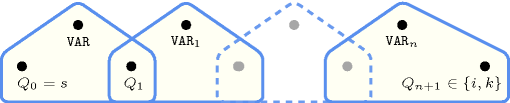## 5.70. clause_or

Origin

Logic

Constraint

$\mathrm{𝚌𝚕𝚊𝚞𝚜𝚎}_\mathrm{𝚘𝚛}\left(\mathrm{𝙿𝙾𝚂𝚅𝙰𝚁𝚂},\mathrm{𝙽𝙴𝙶𝚅𝙰𝚁𝚂},\mathrm{𝚅𝙰𝚁}\right)$

Synonym

$\mathrm{𝚌𝚕𝚊𝚞𝚜𝚎}$.

Arguments
 $\mathrm{𝙿𝙾𝚂𝚅𝙰𝚁𝚂}$ $\mathrm{𝚌𝚘𝚕𝚕𝚎𝚌𝚝𝚒𝚘𝚗}\left(\mathrm{𝚟𝚊𝚛}-\mathrm{𝚍𝚟𝚊𝚛}\right)$ $\mathrm{𝙽𝙴𝙶𝚅𝙰𝚁𝚂}$ $\mathrm{𝚌𝚘𝚕𝚕𝚎𝚌𝚝𝚒𝚘𝚗}\left(\mathrm{𝚟𝚊𝚛}-\mathrm{𝚍𝚟𝚊𝚛}\right)$ $\mathrm{𝚅𝙰𝚁}$ $\mathrm{𝚍𝚟𝚊𝚛}$
Restrictions
 $|\mathrm{𝙿𝙾𝚂𝚅𝙰𝚁𝚂}|+|\mathrm{𝙽𝙴𝙶𝚅𝙰𝚁𝚂}|>0$ $\mathrm{𝚛𝚎𝚚𝚞𝚒𝚛𝚎𝚍}$$\left(\mathrm{𝙿𝙾𝚂𝚅𝙰𝚁𝚂},\mathrm{𝚟𝚊𝚛}\right)$ $\mathrm{𝙿𝙾𝚂𝚅𝙰𝚁𝚂}.\mathrm{𝚟𝚊𝚛}\ge 0$ $\mathrm{𝙿𝙾𝚂𝚅𝙰𝚁𝚂}.\mathrm{𝚟𝚊𝚛}\le 1$ $\mathrm{𝚛𝚎𝚚𝚞𝚒𝚛𝚎𝚍}$$\left(\mathrm{𝙽𝙴𝙶𝚅𝙰𝚁𝚂},\mathrm{𝚟𝚊𝚛}\right)$ $\mathrm{𝙽𝙴𝙶𝚅𝙰𝚁𝚂}.\mathrm{𝚟𝚊𝚛}\ge 0$ $\mathrm{𝙽𝙴𝙶𝚅𝙰𝚁𝚂}.\mathrm{𝚟𝚊𝚛}\le 1$ $\mathrm{𝚅𝙰𝚁}\ge 0$ $\mathrm{𝚅𝙰𝚁}\le 1$
Purpose

Given a first collection of 0-1 variables $\mathrm{𝙿𝙾𝚂𝚅𝙰𝚁𝚂}={U}_{1},{U}_{2},\cdots ,{U}_{p}$, a second collection of 0-1 variables $\mathrm{𝙽𝙴𝙶𝚅𝙰𝚁𝚂}={V}_{1},{V}_{2},\cdots ,{V}_{n}$, and a variable $\mathrm{𝚅𝙰𝚁}$, enforce $\mathrm{𝚅𝙰𝚁}=\left({U}_{1}\vee {U}_{2}\vee \cdots \vee {U}_{p}\right)\vee \left(¬{V}_{1}\vee ¬{V}_{2}\vee \cdots \vee ¬{V}_{n}\right)$.

Example
$\left(〈0,0〉,〈0〉,1\right)$
Typical
$|\mathrm{𝙿𝙾𝚂𝚅𝙰𝚁𝚂}|+|\mathrm{𝙽𝙴𝙶𝚅𝙰𝚁𝚂}|>1$
Symmetries
• Items of $\mathrm{𝙿𝙾𝚂𝚅𝙰𝚁𝚂}$ are permutable.

• Items of $\mathrm{𝙽𝙴𝙶𝚅𝙰𝚁𝚂}$ are permutable.

Arg. properties
• Extensible wrt. $\mathrm{𝙿𝙾𝚂𝚅𝙰𝚁𝚂}$ when $\mathrm{𝚅𝙰𝚁}=1$.

• Extensible wrt. $\mathrm{𝙽𝙴𝙶𝚅𝙰𝚁𝚂}$ when $\mathrm{𝚅𝙰𝚁}=1$.

Remark

The $\mathrm{𝚌𝚕𝚊𝚞𝚜𝚎}_\mathrm{𝚘𝚛}$ constraint is called $\mathrm{𝚌𝚕𝚊𝚞𝚜𝚎}$ in Gecode (http://www.gecode.org/).

Systems
Keywords
Automaton

Figure 5.70.1 depicts the automaton associated with the $\mathrm{𝚌𝚕𝚊𝚞𝚜𝚎}_\mathrm{𝚘𝚛}$ constraint:

• To the argument $\mathrm{𝚅𝙰𝚁}$ of the $\mathrm{𝚌𝚕𝚊𝚞𝚜𝚎}_\mathrm{𝚘𝚛}$ constraint corresponds the first signature variable.

• To each variable of the argument $\mathrm{𝙿𝙾𝚂𝚅𝙰𝚁𝚂}$ corresponds a signature variable.

• Finally, to each variable ${\mathrm{𝚅𝙰𝚁}}_{i}$ of the argument $\mathrm{𝙽𝙴𝙶𝚅𝙰𝚁𝚂}$ corresponds a signature variable that is the negation of ${\mathrm{𝚅𝙰𝚁}}_{i}$.

##### Figure 5.70.1. Automaton of the $\mathrm{𝚌𝚕𝚊𝚞𝚜𝚎}_\mathrm{𝚘𝚛}$ constraint (${\mathrm{𝙿𝚅𝙰𝚁}}_{i}$ and ${\mathrm{𝙽𝚅𝙰𝚁}}_{i}$ respectively denote variables of $\mathrm{𝙿𝙾𝚂𝚅𝙰𝚁𝚂}$ and $\mathrm{𝙽𝙴𝙶𝚅𝙰𝚁𝚂}$)##### Figure 5.70.2. Hypergraph of the reformulation corresponding to the automaton of the $\mathrm{𝚌𝚕𝚊𝚞𝚜𝚎}_\mathrm{𝚘𝚛}$ constraint (${\mathrm{𝚅𝙰𝚁}}_{1},\cdots ,{\mathrm{𝚅𝙰𝚁}}_{n}$ denotes ${\mathrm{𝙿𝚅𝙰𝚁}}_{1},\cdots ,{\mathrm{𝙿𝚅𝙰𝚁}}_{|\mathrm{𝙿𝙾𝚂𝚅𝙰𝚁𝚂}|},1-{\mathrm{𝙽𝚅𝙰𝚁}}_{1},\cdots ,1-{\mathrm{𝙽𝚅𝙰𝚁}}_{|\mathrm{𝙽𝙴𝙶𝚅𝙰𝚁𝚂}|}$)